Compound Growth using DAX - Excelerator BI

# Compound Growth using DAX

There are some things that are very easy to do in Excel that don’t seem to have an immediately obvious and simple solution in DAX.  One such problem is when you want to apply compound growth on an investment.  Let me make up an example so it is clear what I am talking about.  The examples used in this post can be applied to NPV using the XNPV function.

## Simple Example – Fixed Growth Rate

Say you make an investment of \$10,000 in a stock, and you want to forecast what that investment will be worth in 10 years time taking into account a projected annual growth rate.  Here is how you could do that in Excel.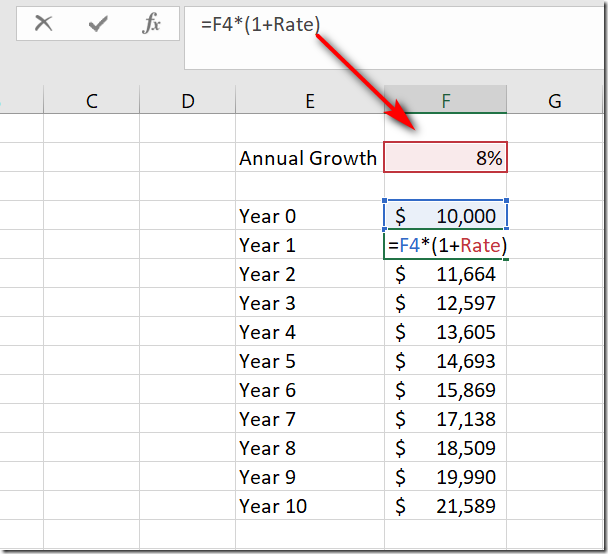Start with the value of the investment in year 0.  Then in all future years, multiply the value from the previous year by (1 + annual growth rate), or 1.08 in the example above.  What makes Excel such an easy tool to use is that you can write one formula for Year 1, then copy it down the page.  Each subsequent formula takes the result from the previous year and adds the growth for the current year to the previous calculation.

For the example above, you can use a simple single cell Excel formula to determine the value in a future year as follows, without having to calculate all the values for each year one at a time.

`=FV(0.08,10,0,-10000)`

The Function above applies an investment rate of 8%, over 10 years, making 0 extra payments, on an initial investment of \$10,000.  This simple formula returns the same value of \$21,589 in year 10; same as in the  Excel example above.

## Harder Example – Variable Growth Rate

It is harder to write a single cell formula/function when you have a table of growth rates that vary over time.  But this harder example is still quite simple in a regular Excel workbook as shown below.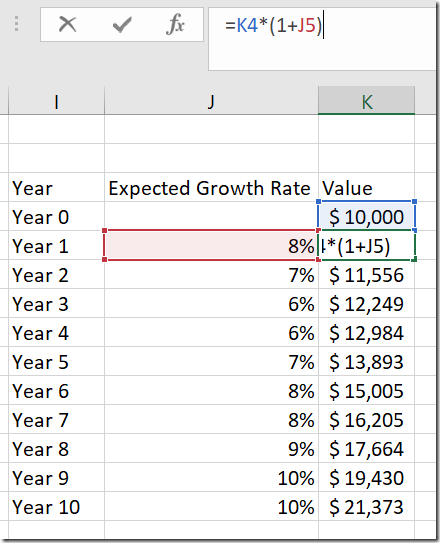The formula is still essentially the same as the first Simple Excel example at the start of this article, but this time the calculation uses the forecasted rate for each year in the year by year calculation rather than a fixed annual rate.

## Compound Growth Using DAX

Now back to the point of this article.  Compounding growth is very easy to do in Excel because you can write individual cell formulas (to do what ever you want), and each new formula can reference the answer from the previous formula as the starting point for the new formula. There is no such ability in the DAX language.  To solve such problems in DAX, you have to change the way you think and start to think about how to write a single formula that will work over an entire TABLE of data (or columns or multiple tables) – no cell by cell individual formulas are possible.

Below I will step you through the process of finding a solution to this problem.  As I often mention in my articles, it is the process that I believe is most important.  I seldom know how to answer a complex DAX problem when I start out (that’s the very definition of “complex”), and instead I follow a process to help me solve the problem.  Take a careful read below.  If you apply the same process when you write your formulas, you will be well on your way to becoming a DAX Superhero.

### Step 1 – Load The Data

Because I am already in Excel, I loaded up the sample data from the example above into Power Pivot for Excel 2016.  The results would be the same if I solved the problem using Power BI Desktop.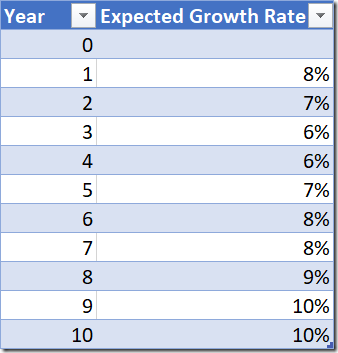Note that I have loaded up the year numbers as Integers instead of the text “Year 1, Year 2” etc.  This is an essential part of the solution to this problem, having an ordinal index indicating the order of the rows in the table.

### Step 2 – Create a Pivot Table

This is one of my most important tips ever, and you would be well advised to take heed.  Before you do anything else, create a pivot table so you can “see” your data that lives in the underlying table.  This is particularly true for Excel folk (like me) as we are used to “seeing” stuff that we are working on.  It is orders of magnitude harder to write complex DAX formulas when you are not looking at a sample of your data on the screen.  If you are writing DAX in Power BI Desktop, I recommend you add a Matrix to the report and use that as the starting point.

Here is my pivot table, in tabular format, with subtotals turned off and the Year and Rate both placed on Rows.  I have written a simple measure Initial Investment = 10000 and placed that on Values in the pivot table.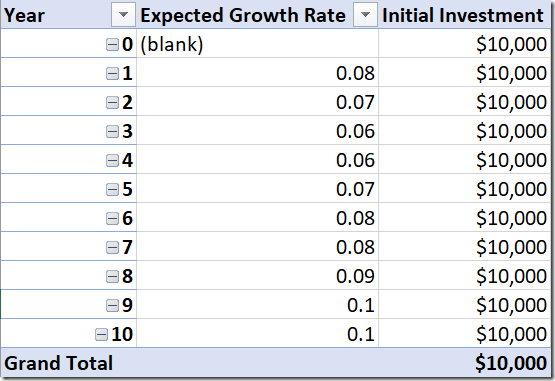### Step 3 – Thinking Through the Problem

Now that you can “see” the data coming from the table in the pivot table above, it is easier to think through the problem.  Just as a reminder on the “challenge” at hand; we need to be able to write a single formula that will give the correct result for every row in the table – there is no opportunity to do cell by cell calculations and no opportunity to “point” to the previous result and include that inside any formula.

The second concept you need to get your head around is thinking of your data as “tables of data with filters applied (or not)”. There can only be a single formula that gives the correct results, and each result will be different due to the different filters that will be applied to the underlying data table.  With that in mind, let me show you what we need as the formula to calculate the future value as at any single year.

Year 0 = 10,000 * (1+ 0.00)

Year 1 = 10,000 * (1+ 0.00) * (1+0.08)

Year 2 = 10,000 * (1+ 0.00) * (1+0.08) * (1+0.07)

Year 3 = 10,000 * (1+ 0.00) * (1+0.08) * (1+0.07) * (1+0.06)

Year 4 = 10,000 * (1+ 0.00) * (1+0.08) * (1+0.07) * (1+0.06) * (1+0.06)

… and so on.

So you should identify the pattern above as “for each year, multiply the initial investment by (1 + growth rate) for each year up until the current year.  Referring to my tip above, is should be clear now that you need to filter the table of data so that you can access the current year and all the years prior to complete the calculation.  That is starting to look like a standard FILTER pattern to me.### Step 4 – Write an Interim Test Measure

The next thing to do is to write an interim test measure that “tests out” a filter pattern that keeps every row in the table up to and including the current row.  The final result of the test measure I wrote is shown below; it returns a count of how many rows are filtered for each row of the pivot table.

```FilteredRows =
VAR LatestYear = MAX(Rates[Year])
VAR UnfilteredTable = ALL(Rates)
RETURN
COUNTROWS(FILTER(UnfilteredTable, Rates[Year] <= LatestYear))
```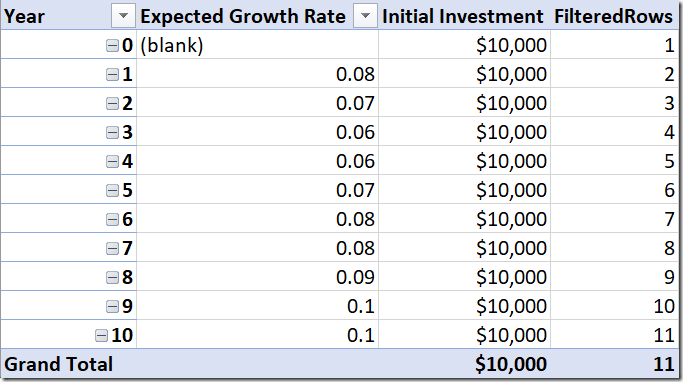Test measures like this are great to help you work through the problem by breaking the problem down into pieces and allowing you to “see” the results as you proceed.  I can “see” above from my test measure that the filtered table is growing as it works down the pivot table.  Also I have used the VAR syntax to write my measure.  I am increasingly writing my measures this way as it makes the formulas almost self documenting and easier for others to read and understand.  By self documenting, I mean that the variable names are descriptive of what each piece of the formula does, and that makes the formula easier to understand.### Step 5 – Write the Final Measure

The final measure needs to multiply the initial investment by (1 + growth rate) for each row in the table after the filter has been applied.  That sounds like an iterating X function!  I know there is a SUMX, MINX, MAXX etc, so I went searching (using Intellisense) for a MULTIPLYX and TIMESX, but I lucked out.  I then remembered my DAX Quick Reference Guide that I prepared a few years ago – you can download a copy of it for free here.  A quick look in the PDF and I found what I was looking for – PRODUCTX. This function was released in Excel 2016 and Power BI Desktop, so no luck if you are using Excel 2013 or earlier.

Here is my final formula (reusing the guts of the interim test measure from above).

```Future Value =
VAR LatestYear = MAX(Rates[Year])
VAR UnfilteredTable = ALL(Rates)
RETURN
[Initial Investment]
* CALCULATE(
PRODUCTX(Rates, 1+Rates[Expected Growth Rate]),
FILTER(UnfilteredTable, Rates[Year] <= LatestYear)
)
```

And here are the results of this measure in my pivot table (same answers as the Excel version at the top of the page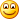).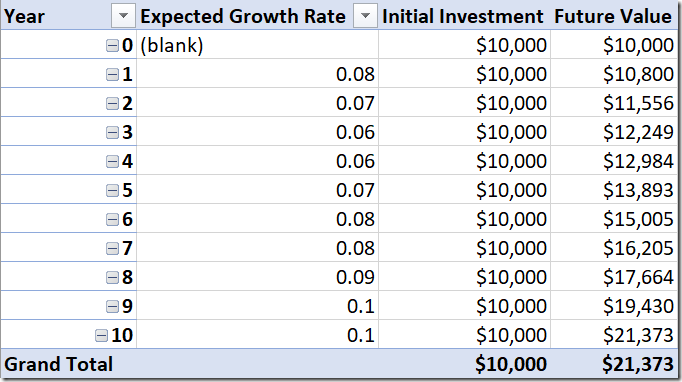The way the formula above works is

First the filter is applied to the table, so only the rows that should be included remain in the filter context. Then the PRODUCTX function iterates over this filtered table, one row at a time, multiplying (1+rate) for every row in the filtered table.  Finally this compound factor is multiplied by the initial investment.

You can also read my article on User Specified Growth on an Investment using Power BI.

### 30 thoughts on “Compound Growth using DAX”

1.Does it work with MEASURE?

I have created a Running Total Measure, but can I use that to calculate compound growth?

2.That was super informative and I love how you broke down the process vs just show a solution.

3.Hey Matt. This is a really useful usecase for ProductX. I’m trying to implement something like this in PowerBI, but one thing I cannot get around is that my expected growth rate value is a measure in my model rather than a column.

As far as I can tell ProductX only accepts columns for its second argument and not measures- and I’m finding it difficult to work around this issue – is there a way to do this when using expected growth rate as a measure?

1.Interesting question. Technically PRODUCTX steps through a table, one row at a time (that’s the X part), and for each row in the table it completes the formula to return the result. At the end, it multiplies all the results together (that’s the PRODUCT part). There is no reason why a measure cannot be in the “formula” section. For example, if I had an arbitrary table with 3 rows and 1 column, with values A, B C called table. I also have a measure with the value 2. then this formula
PRODUCTX(Table,[Measure]) would return 8. 3 steps where the answer is 2, all multiplied together. So to solve your problem, you need to apply this thinking.

Not sure if that helps or not

1.It does help my thinking Matt – so many thanks for the response. I’m still a rank beginner at PowerBI and still learning some of these iterating functions.

I’ve been trying to get something like what you have in your blog post to work, but I believe it fails when I use a calculated measure.

The ProductX formula syntax does specifically mention that it takes a column as argument 2 (https://docs.microsoft.com/en-us/dax/productx-function-dax)

PRODUCTX(Rates, 1+Rates[Expected Growth Rate])

…if I was to adhere to the formulas rules, [expected growth rate] must be a column , or an expression that evaluates to a column. Unfortunately, I have it as a measure – and I think that’s why it’s not working for me.

1.The documentation is wrong :-). Trust me, you don’t need to refer to a column in the expression.

I just reported the error https://github.com/MicrosoftDocs/feedback/issues/3571

1.Thanks Matt – and good to know it wasn’t me 🙂

Clearly I think I have something else going on and its probably model related. I’ll keep hacking away until I get it right 🙂

4.I have 2 tables, cash & assets. I made a measure: (current months’s assets – current month’s cash)/previous month’s assets. It gives me a % return, which is great. However, I’m trying to get a running return by month but nothing is working. I’ve tried the ProductX on this % measure, but it comes up blank. I’ve also tried the Calc(ProductX…Filter(…<=Max as mentioned above, but it comes up blank as well. Any ideas how to get ProductX to work on this measure?

1.These can be tricky little suckers. I can’t really help you properly without seeing what is going on. If you can re-create the problem in a non-sensitive dataset, I suggest you ask at community.powerbi.com. But for some general advice, the answer is ALWAYS filtering. The trick is to create a visual (I usually use a matrix or table) and layout the data so you can see what is going on. Break the problem into it’s component parts so you can see which bit is working and which is not. Ask yourself, “what filters are preventing me seeing what I expect to see”.

You can also engage me for commercial support if you like by purchasing an hour of time here http://xbi.com.au/matt

5.Brandon Stevens

How can I search your blog posts to find the one on 9th July 2018?

6.How can this be scaled up? In my requirements i have multiple instruments in the same table with varying growth projections and when applying the above formula the growth rate for each instrument sums and applies to all.

1.It is a hard question as I don’t have a clear picture of what you have in front of you. You could post some samples and ask for help on community.powerbi.com I guess.

7.Sorry B, this is too hard to answer from what you have provided. If you set up a sample Excel file showing how it works in Excel, and suitable data loaded into the data model, then post this on http://powerpivotforum.com.au I will take a look when I get a moment.

8.How would you solve for this scenario but with periodic investments? So the EOY balance is balance from last year + interest + period investment, etc? I can do this in Excel in 20 seconds, but I’m stuck in DAX to compute this in a measure to plot future balances on a line chart.

1.Dawn Christenson

I have this exact same problem and have not found a solution anywhere. Is DAX not able to do this?

9.This is an excellent solution and painfully close to what I need. Is there a way to allow users to select (say via slicer) from a list of flat-rate growth-rate multipliers (say 1.01, 1.02,… 1.10) that will then be applied to each forecast year while still compounding properly just as this solution does?

1.10.Duncan Williamson

Matt,
For a reason I cannot fathom, when I run the FilterRows code, every row returns 11. I thought, that’s me for ya! So I ignored it and then applied the code for the Future Value column: every row shows 21,372.85 … I get the same answers with Oscar’s code too.

Any ideas?

1.can you email me the workbook you are using?

1.Duncan Williamson

OK, on its way

Thanks

Duncan

11.Very good Matt. Thanks for your input. Every time I learn more with your posts. You might as well have written it the following way, right?

= VAR
LatestYear = MAX (rates [Year])
Var
UnFilteredTable = ALL (rates)
RETURN
PRODUCTX (
FILTER (UnFilteredTable; Rates [Year] < = LatestYear);
(1 + rates [Expected Growth rate])
) * [Initial Investment]

1.Yes, there are many ways to write formula and your way is good. One thing to note is that if it were a multi table model, it may be necessary to use CALCULATE, but only if context transition is needed to complete the task (I’m not saying it would be needed, just sayin’).

2.Duncan Williamson

Oscar, in line 7 of your code I had to change ; to , … that’s probably due to my international settings, yes?

12.Good day, Have problem with Measure in place ” [Initial Investment] * CALCULATE (” (The value for ‘Initial Investment’ cannot be determined. Either ‘Initial Investment’ doesn’t exist, or there is no current row for a column named ‘Initial Investment’.) Only CALCULATE part works well.

1.Did you write the measure [Initial Investment] ?

13.Thankyou Matt and thankyou Andrew. I can’t wait to try them. Excel is powerful. However, trying to replicate excel formulas in DAX can be torturous as you pointed out and as I’ve discovered trying to re-engineer Exel solutions.

14.Hi Matt
Great post.
Firstly, here is a formula that works with Excel 2013.
[DAX]
Future value XL2013:=[Initial investment]*
CALCULATE(
EXP(SUMX(Rates,LN(1+Rates[Expected growth rate]))),
FILTER(All(Rates),Rates[Year]<=MAX(Rates[Year])))
[/DAX]
It uses the natural log to turn the sum of values into the product of values.

Secondly, I think the below formula for the will be more efficient for PBI. It takes advantage of the fact declaring a variable enables the filter function be avoided (my understanding is that the filter is an iterator function and therefore very slow).
[DAX]Future value =
VAR LatestYear=MAX(Rates[Year])
RETURN
[Initial investment]*
CALCULATE(
PRODUCTX(
Rates,
1+Rates[Expected growth rate]),
Rates[Year]<=LatestYear)
[/DAX]

Interested to hear your thoughts on avoiding the filter function.

1.Nice Excel 2013 alternate! Regarding FILTER being inefficient because it is an iterator; this is not a blanket correct statement. Filter is an iterator, but under the hood there are engine optimisations that can and do achieve the outcomes more efficiently than is implied by the concept of iteration. I would generally advise against using a Filter over a 10 million row table, but it is fine to iterate over a low cardinality column in the same table. Also, your suggested formula doesn’t avoid FILTER at all.
This
1.